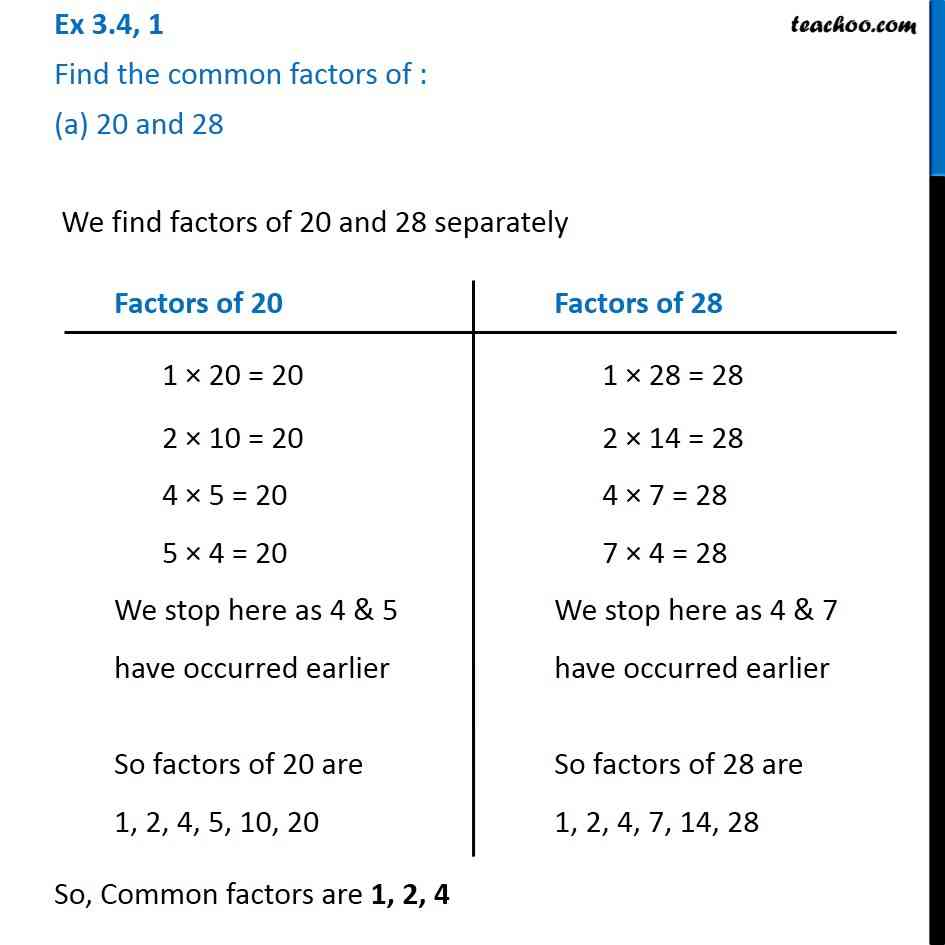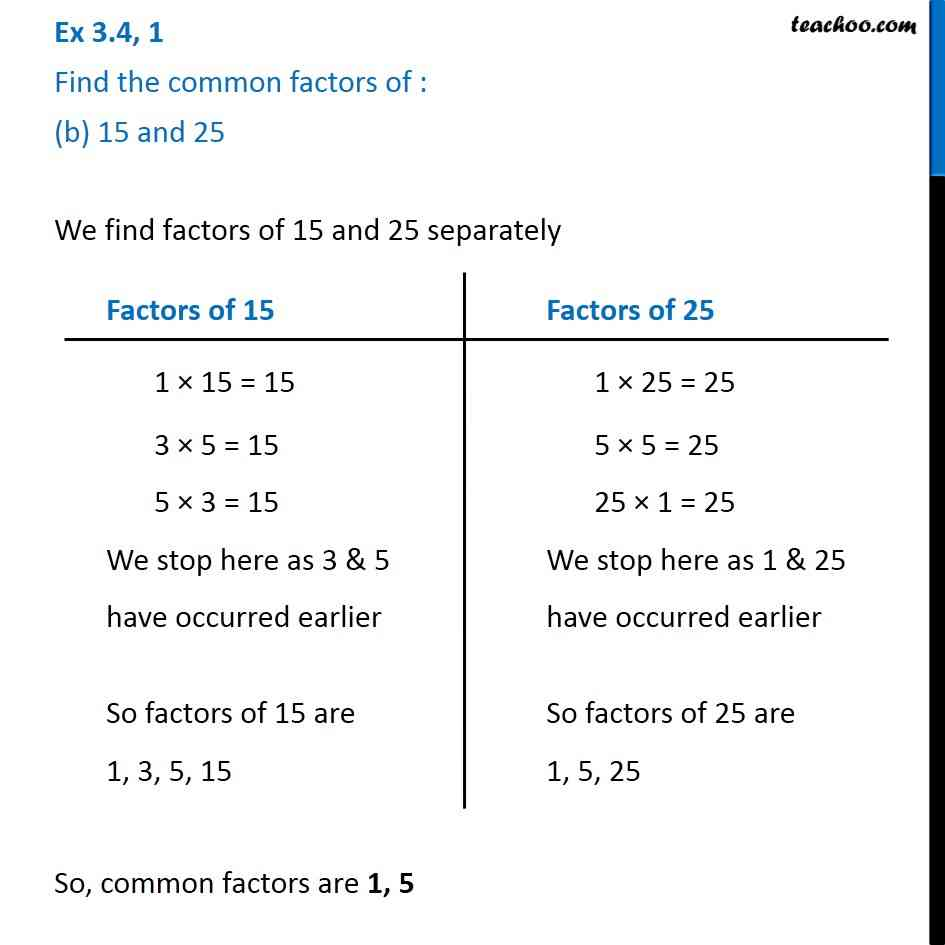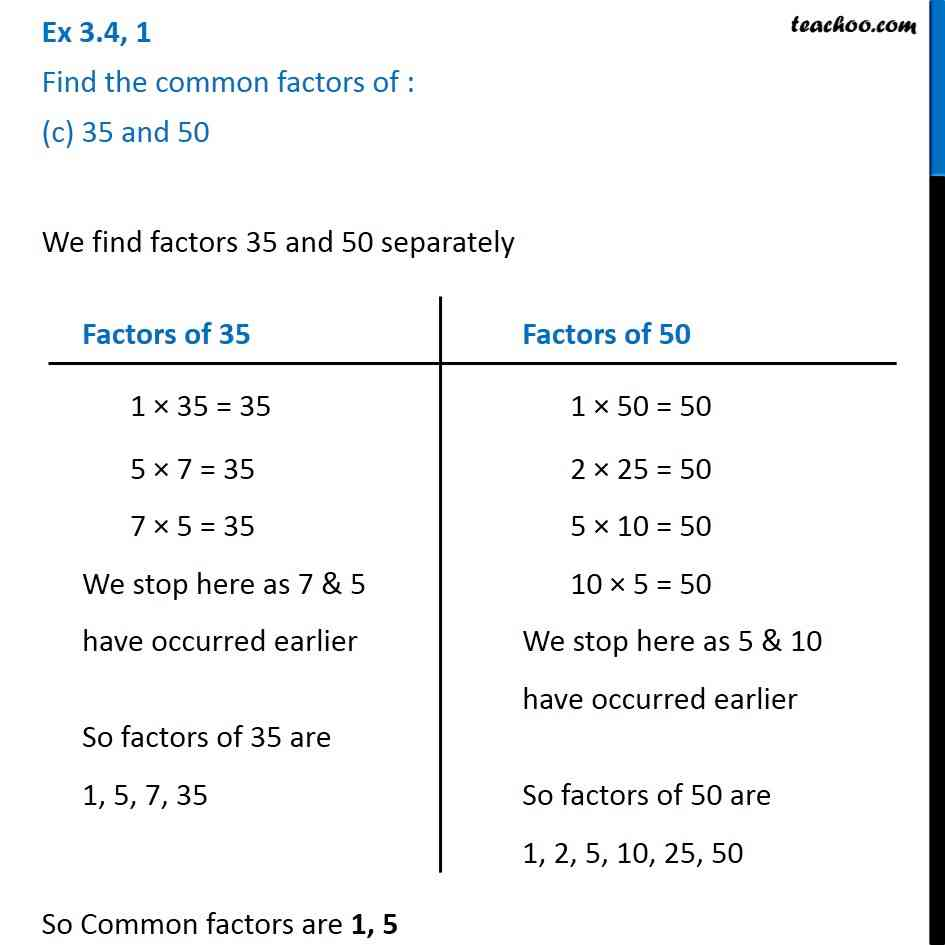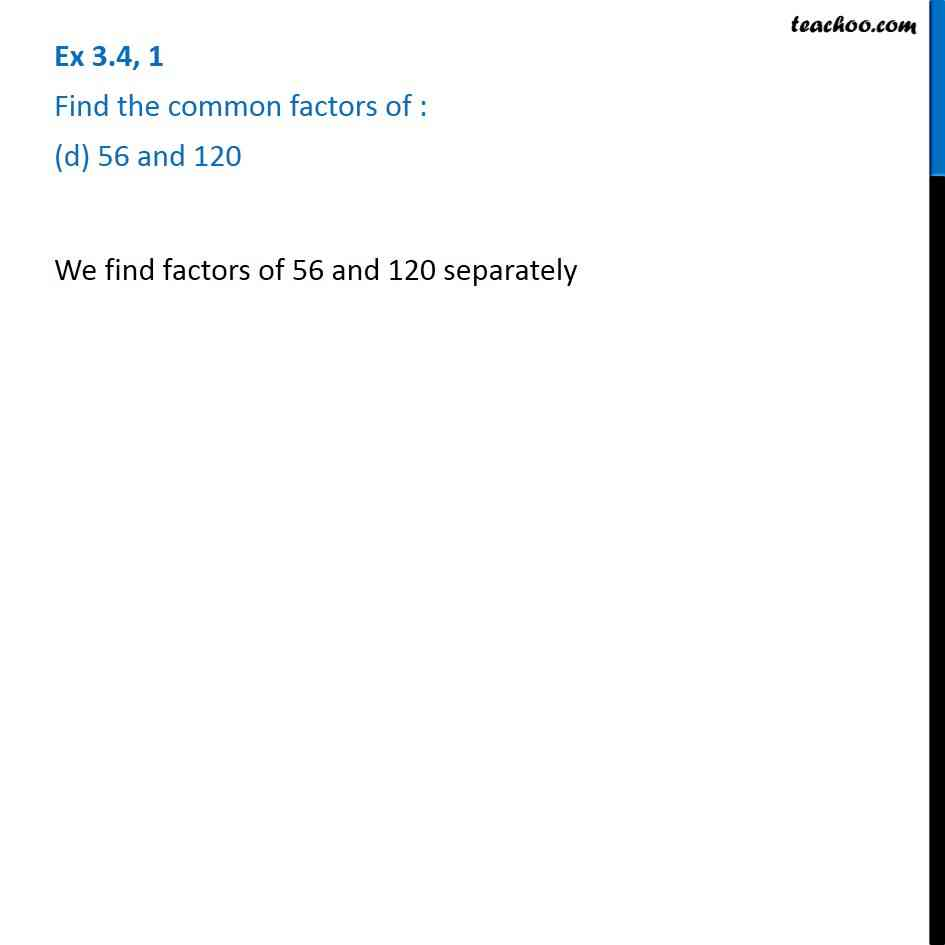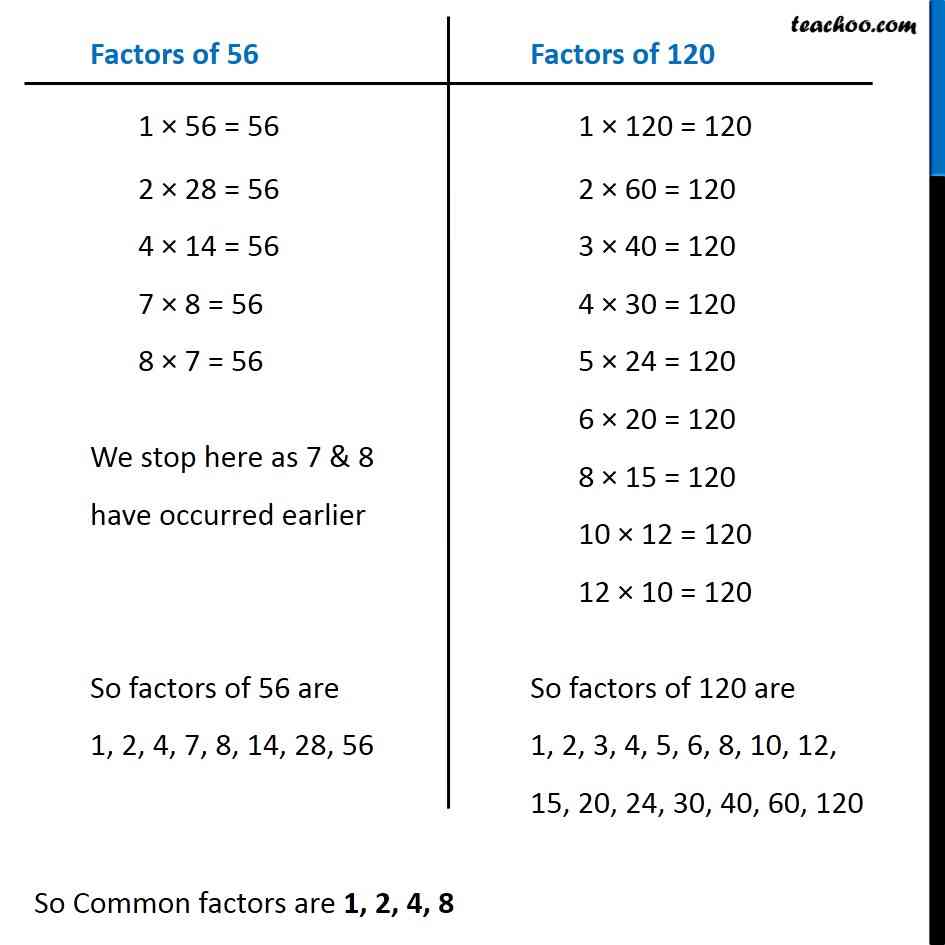Subscribe to our Youtube Channel - https://you.tube/teachoo

1. Chapter 3 Class 6 Playing with Numbers
2. Serial order wise
3. Ex 3.4

Transcript

Ex 3.4, 1 Find the common factors of : (a) 20 and 28 We find factors of 20 and 28 separately So, Common factors are 1, 2, 4 Factors of 20 1 × 20 = 20 2 × 10 = 20 4 × 5 = 20 5 × 4 = 20 We stop here as 4 & 5 have occurred earlier So factors of 20 are 1, 2, 4, 5, 10, 20 Factors of 28 1 × 28 = 28 2 × 14 = 28 4 × 7 = 28 7 × 4 = 28 We stop here as 4 & 7 have occurred earlier So factors of 28 are 1, 2, 4, 7, 14, 28 Factors of 28 1 × 28 = 28 2 × 14 = 28 4 × 7 = 28 7 × 4 = 28 We stop here as 4 & 7 have occurred earlier So factors of 28 are 1, 2, 4, 7, 14, 28 Factors of 28 1 × 28 = 28 2 × 14 = 28 4 × 7 = 28 7 × 4 = 28 We stop here as 4 & 7 have occurred earlier So factors of 28 are 1, 2, 4, 7, 14, 28 Factors of 28 1 × 28 = 28 2 × 14 = 28 4 × 7 = 28 7 × 4 = 28 We stop here as 4 & 7 have occurred earlier So factors of 28 are 1, 2, 4, 7, 14, 28 Ex 3.4, 1 Find the common factors of : (b) 15 and 25 We find factors of 15 and 25 separately So, common factors are 1, 5 Factors of 15 1 × 15 = 15 3 × 5 = 15 5 × 3 = 15 We stop here as 3 & 5 have occurred earlier So factors of 15 are 1, 3, 5, 15 Factors of 25 1 × 25 = 25 5 × 5 = 25 25 × 1 = 25 We stop here as 1 & 25 have occurred earlier So factors of 25 are 1, 5, 25 Ex 3.4, 1 Find the common factors of : (c) 35 and 50 We find factors 35 and 50 separately So Common factors are 1, 5 Factors of 35 1 × 35 = 35 5 × 7 = 35 7 × 5 = 35 We stop here as 7 & 5 have occurred earlier So factors of 35 are 1, 5, 7, 35 Factors of 50 1 × 50 = 50 2 × 25 = 50 5 × 10 = 50 10 × 5 = 50 We stop here as 5 & 10 have occurred earlier So factors of 50 are 1, 2, 5, 10, 25, 50 Ex 3.4, 1 Find the common factors of : (d) 56 and 120 We find factors of 56 and 120 separately Factors of 56 1 × 56 = 56 2 × 28 = 56 4 × 14 = 56 7 × 8 = 56 8 × 7 = 56 We stop here as 7 & 8 have occurred earlier So factors of 56 are 1, 2, 4, 7, 8, 14, 28, 56 Factors of 120 1 × 120 = 120 2 × 60 = 120 3 × 40 = 120 4 × 30 = 120 5 × 24 = 120 6 × 20 = 120 8 × 15 = 120 10 × 12 = 120 12 × 10 = 120 So factors of 120 are 1, 2, 3, 4, 5, 6, 8, 10, 12, 15, 20, 24, 30, 40, 60, 120

Ex 3.4